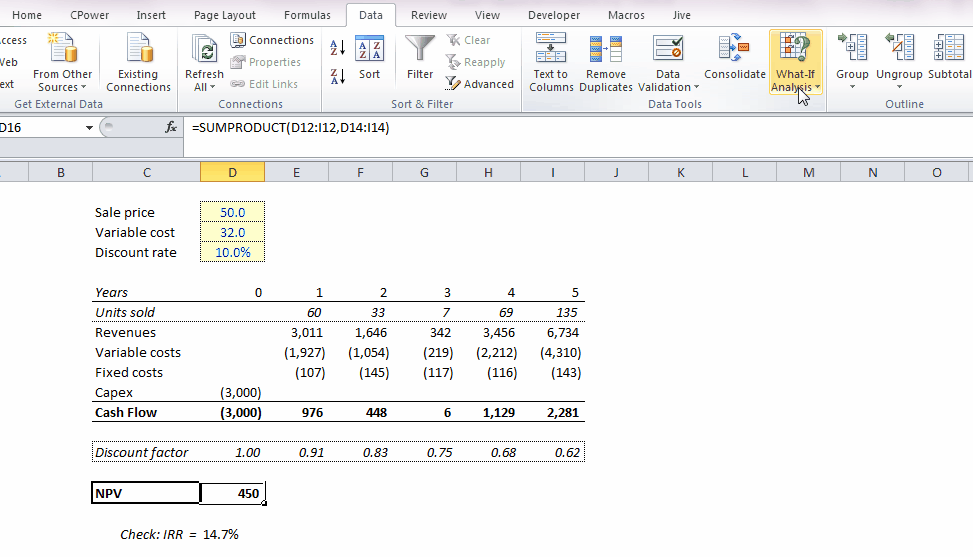top of page

Goal Seek

## The Goal Seek function in Excel allows you to ask Excel to find a specific result you want by adjusting an input value.

Excel modeling

1. Calculate the result you want to analyze (NPV, IRR, etc)

2. Go to Data / What-If Analysis / Goal Seek

3. In "Set Cell" choose the cell that contains the output of the model you want to analyze

4. In "To value" choose the value that yo want the output cell to be equal to

5. In "By changing cell" indicate to Excel what is the variable that can be adjusted to end up with the desired result

In the example below, the user wants to calculate what the sale price of the product be such that the NPV of the project is equal to 0.bottom of page